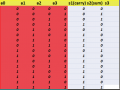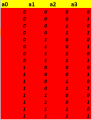#### Leite33

Joined Nov 28, 2015
57
Dear Friends
I want your help again. I have to create as a homework one simple circuit that should have 4 inputs x0,x1,x2,x3 and the output will be a number with 3 binary digit like s2s1s0 that will be the sum Σ=x0+x1+x2+x3 . I have to use to do the circuit only adders and semiadders. Can anyone help?
Also i want to ask what is the most useful program to create circuits. I use microsoft visio.

#### WBahn

Joined Mar 31, 2012
28,520
There are tons of ways to draw schematics. Many of the schematics I've posted here I've just done using Paint. I use to do them with AutoCAD and then PowerPoint. Visio has some libraries but I never found it too friendly because it always wanted to do my thinking for me. But you should move to using a program that is specifically intended for schematics, so that then you can start simulating them and getting board netlists and bills of material. You can use a simulator that has schematic capture, such as LTSpice. Or you can use a schematic capture program such as SchemeIt from DigiKey. The first is downloadable and the second is only online. SchemeIt does very nice schematics and is pretty easy to use. There are dozens of other options, too. Many of them free.

As for your homework question, remember that it is YOUR homework. YOU need to show YOUR best attempt to solve YOUR homework. We can then look your work over and give hints and suggestions to help lead you in the right direction.

#### Leite33

Joined Nov 28, 2015
57
Thanks friend i found Schemelt very usefull. As for the homework i try to understand somethings and make a start. I think forums is very useful to learn things

#### Leite33

Joined Nov 28, 2015
57
I am confused here too. And i have the same problem i try to do the truth table. I have a0,a1,a2,a3 as inputs but i dont understand the outputs. s2,s1,s0. We said that adder make the sum of the inputs. So the one output will be the sum, the other output will be the carry and what about the third output?? I need here an example too of truth table so to make a start.So the s2 sum is 1 when only one input is 1 or all inputs(4) are 1. The output carry S1 is 1 when the 2 or 3 outputs are 1. * What about if 4 inputs are 1 i guess S1 is 1 too. And what about the third output S3

#### WBahn

Joined Mar 31, 2012
28,520
The output is a three-bit binary number that equals the sum of the four one-bit binary numbers that are inputs.

If the inputs are all 1, what is the sum of them? How is this represented as a three-bit binary number?

#### hp1729

Joined Nov 23, 2015
2,304
Dear Friends
I want your help again. I have to create as a homework one simple circuit that should have 4 inputs x0,x1,x2,x3 and the output will be a number with 3 binary digit like s2s1s0 that will be the sum Σ=x0+x1+x2+x3 . I have to use to do the circuit only adders and semiadders. Can anyone help?
Also i want to ask what is the most useful program to create circuits. I use microsoft visio.
Re: schematic software

I use ORCAD. The free version is suitable for a page or two per schematic, about 60 symbols. The library of parts is extensive. Any part not in the library you can create with a little practice. The full version lets you create a pc board and a parts list from a schematic. No limit on number of schematics or such. I've been using the free versions for a decade or so and get upgrades every few years.
It has pinouts for different packages of a given part.

#### Leite33

Joined Nov 28, 2015
57
Dear Wbanh
I still try to understand to move on. The one output will lets S2 is the sum of the 4 outputs am i right? The second output lets say S1 is the output of carry am i right? and what about the third output lets say S0? is sum again? i have already sum output? I think after so many hours i cant solve even simple things

#### hp1729

Joined Nov 23, 2015
2,304
The output is a three-bit binary number that equals the sum of the four one-bit binary numbers that are inputs.

If the inputs are all 1, what is the sum of them? How is this represented as a three-bit binary number?
1 + 1 + 1 + 1 = 4 (100)
Are you sure this is what you want? In your other post it is labeled "2-bit full adder" which suggests summing two 2-bit values.
11 (3) + 11 (3) = 110 (6)

In your "simplify this circuit" post this doesn't change the outcome of the simplification either way. In both examples the only time you get 000 out is with 0,0,0,0 in. The output of the OR gate will only be low when all the inputs to the adder are low. (And there is the key to the simplification.)

#### WBahn

Joined Mar 31, 2012
28,520
Dear Wbanh
I still try to understand to move on. The one output will lets S2 is the sum of the 4 outputs am i right? The second output lets say S1 is the output of carry am i right? and what about the third output lets say S0? is sum again? i have already sum output? I think after so many hours i cant solve even simple things

the output will be a number with 3 binary digit like s2s1s0 that will be the sum Σ=x0+x1+x2+x3
The three output bits form a single 3-bit binary number!

Do you understand what a binary number is? If asked to write the decimal number 5 in binary, do you know how to do it?

#### Leite33

Joined Nov 28, 2015
57
Ok i think we have to start from the begging because i am really confused here. "Design an adder that should have 4 binary digits as inputs a0,a1,a2,a3 and will give as an input one 3 digit binary number that will be equal with the sum=a0+a1+a2+a3. To design the circuit use only full adder and semi adder. Now i have to start by creating my truth table.
So far so good i think. Now in my truth table i have 16 number in every column because it says ("4 binary digit "). Am i right? and 4 columns because i have 4 inputs. I am really sorry i am new on that and i try to clear some things on my head.so far so good? Now i have to create my outputs. Ofcourse when i have a0=1 a1= a2=1 a3=1. I get 100 so my outputs will be 1 0 0 in that row? what about the output that will give my carry? Do i need it? The exercise says " will give one 3digit binary number s2s1s0". Maybe my output is one (for example 100) depends on the values mixed of s0s1s2 and is more simple than i think. I need help to make the truth table and move on with the circuit.
5 is 101 in binary

#### WBahn

Joined Mar 31, 2012
28,520
The problem description says nothing about any kind of carry output. It is asking for a single output that consists of three bits where those three bits are a three-bit binary number that represents the sum of all four of the one-bit binary inputs.

#### hp1729

Joined Nov 23, 2015
2,304
Ok i think we have to start from the begging because i am really confused here. "Design an adder that should have 4 binary digits as inputs a0,a1,a2,a3 and will give as an input one 3 digit binary number that will be equal with the sum=a0+a1+a2+a3. To design the circuit use only full adder and semi adder. Now i have to start by creating my truth table.
So far so good i think. Now in my truth table i have 16 number in every column because it says ("4 binary digit "). Am i right? and 4 columns because i have 4 inputs. I am really sorry i am new on that and i try to clear some things on my head.
View attachment 96003
so far so good? Now i have to create my outputs. Ofcourse when i have a0=1 a1= a2=1 a3=1. I get 100 so my outputs will be 1 0 0 in that row? what about the output that will give my carry? Do i need it? The exercise says " will give one 3digit binary number s2s1s0". Maybe my output is one (for example 100) depends on the values mixed of s0s1s2 and is more simple than i think. I need help to make the truth table and move on with the circuit.
5 is 101 in binary
Is the adder just supposed to sum four 1-bit binary values and get a result of a three-bit binary value?
0 + 1 + 1 + 1 = 3 (011)

In the "simplify the circuit" question this is made meaningless by the OR gate at the output. Any high out of the adder results in a high out of the OR gate. Only all lows into the adder gets a low out of the OR gate. This simplifies the "adder and OR gate" to just being a four-input OR gate. Any high in gets a high out.

7

#### hp1729

Joined Nov 23, 2015
2,304
True, no carry.

Regarding the big circuit. The output of the adder doesn't matter except that zeros in gets zeros out. Any combination of ones in gives a high out. Specifics don't matter. The adder and OR gate simplifies to a four input OR gate.
The next question is what will the inputs to the adder be like?

#### WBahn

Joined Mar 31, 2012
28,520
Keep in mind that we don't know for sure that this problem and "the big circuit" are related. My guess is that this is a separate problem but that the result of this one is used in "the big circuit". But we don't really know that for sure. It's best if we try to keep a thread focused on the problem of that thread -- if for no other reason than someone reading this thread has no idea what is meant by "the big circuit".

#### WBahn

Joined Mar 31, 2012
28,520
Yes, your truth table is finally right.

But what is this "array" you are now talking about?

#### WBahn

Joined Mar 31, 2012
28,520
First off (and I didn't spot this on your last post), your output signals are s2,s1,s0 with s2 being the msb and s0 being the lsb.

Your maps (what are called Karnaugh maps, or just K-maps) are specifically intended to help you come up with minimal SOP or POS equations for logic function. If you don't know how to use them, then open that textbook again and look. Then make your best attempt and we can go from there.

Are you allowed to use XOR gates?

#### Leite33

Joined Nov 28, 2015
57
Well i allowed to use only full adder and semi adder. Well in the first k-map i have only 1 n the center and i am not sure at all.
s1=a0a1'a2a3
So is this from the first karnaugh map right? Do i really need them?

Last edited:

#### WBahn

Joined Mar 31, 2012
28,520